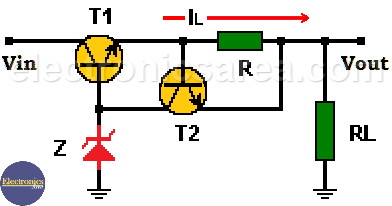# Current Limiter for Voltage Regulator with transistor and Zener diode

When the amount of current required of from a power supply exceeds its maximum capacity, we need a Current Limiter. (Over current protection)

The voltage regulator we use is made up of a zener diode and a pass-through transistor. If the current required in the load exceeds the maximum collector current of the transistor, the transistor can be damaged. To avoid this problem it is necessary to have a circuit that protects against over currents.

## How the Current Limiter (Over current protection) works?

The circuit shown, limit the current to a safe level. We have included a transistor and an additional resistor to the Transistor – Zener Voltage regulator (original circuit). When the voltage regulator is working, the current that passes through the load, also passes through the resistor R.

The voltage across the resistor R is: VR = I x R (Ohm’s law), and it is the same voltage of the base-emitter junction of transistor T2. The resistor R has a preset fixed value. The only situation that can make the VR voltage change, is a change on the load current that pass through the resistor R.While the voltage across the resistor is below 0.7 volts, the transistor T2 is off and the voltage source works normally. If there is an increase of the load current (IL), the voltage drop across resistor R increases and when it reaches 0.7 volts, the transistor T2 start to conduct.

The collector of transistor T2 is connected to the base of transistor T1, which is the pass transistor of the regulator.

When the electric current in the load rises above a maximum value, the transistor T2 begins to conduct and takes a bit of the transistor T1 base current, this in turn reduces the collector current IL (load current).

Note: Remember that Ic = β Ib. For a fixed β, if we decrease the base current (Ib), we decrease the collector current (Ic)

We can design a voltage regulator circuit like this for a defined maximum current load.

For example:

1- We want to design a Voltage Regulator with current limiter. The maximum allowable current is 0.5 amps.

We know that the base-emitter voltage (Vbe) of transistor T2 is 0.7 volts and the allowed maximum current is 0.5 amps. The resistor to be used, to achieve our goal is:

R = Vbe / ILmax = 0.7 V / 0.5 Amps = 1.4 ohms. We can use a 1.5 ohm resistor.

The power of the resistor is: (Joule’s Law)

P = I2 x R = 0.52 x 1.5 = 0.375 watts. We can use a 1.5 ohm, ½ watt resistor.

2- We want to design a Voltage Regulator with current limiter. The maximum allowable current is 2 amps.

We know that the base-emitter voltage (Vbe) of transistor T2 is 0.7 volts and the allowed maximum current is 2 amps. The resistor to be used, to achieve our goal is:

R = Vbe / ILmax = 0.7 V / 2 Amps. = 0. 35 ohms. We can use a 0.33 ohm resistor.

The power of the resistor is: (Joule’s Law)

P = I2 x R = 22 x 0.33 = 1.32 watts. We can use a 0.33 ohm, 2 watt resistor.

• 2
•
• 1
•
•
•
•
•
3
Shares
•
3
Shares
• 2
•
• 1
•
•
•
•